# Value never assigned to error#### netarchitech

##### Well-known member
VIP
Given the following: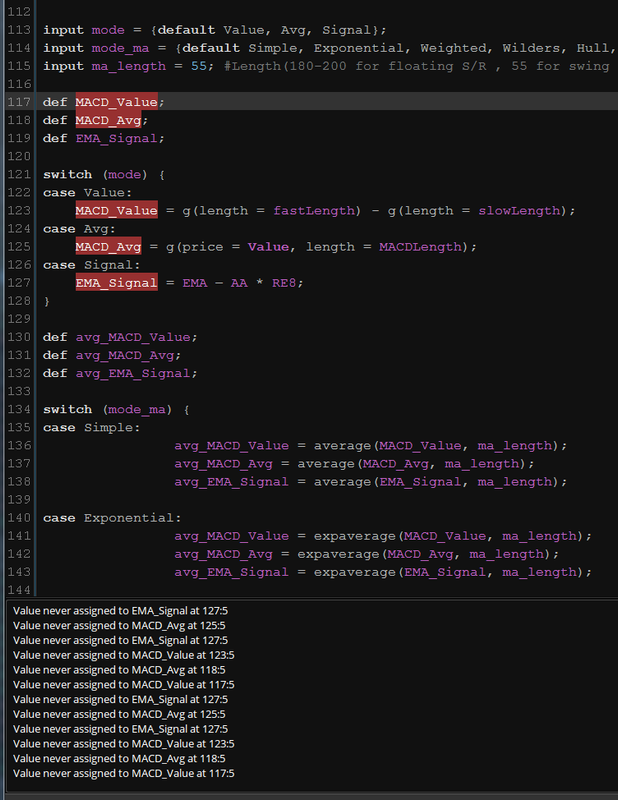Code:
``````input mode = {default Value, Avg, Signal};
input mode_ma = {default Simple, Exponential, Weighted, Wilders, Hull, EHMA, THMA};
input ma_length = 55; #Length(180-200 for floating S/R , 55 for swing entry)

def MACD_Value;
def MACD_Avg;
def EMA_Signal;

switch (mode) {
case Value:
MACD_Value = g(length = fastLength) - g(length = slowLength);
case Avg:
MACD_Avg = g(price = Value, length = MACDLength);
case Signal:
EMA_Signal = EMA – AA * RE8;
}

def avg_MACD_Value;
def avg_MACD_Avg;
def avg_EMA_Signal;

switch (mode_ma) {
case Simple:
avg_MACD_Value = average(MACD_Value, ma_length);
avg_MACD_Avg = average(MACD_Avg, ma_length);
avg_EMA_Signal = average(EMA_Signal, ma_length);

case Exponential:
avg_MACD_Value = expaverage(MACD_Value, ma_length);
avg_MACD_Avg = expaverage(MACD_Avg, ma_length);
avg_EMA_Signal = expaverage(EMA_Signal, ma_length);

case Weighted:
avg_MACD_Value = wma(MACD_Value, ma_length);
avg_MACD_Avg = wma(MACD_Avg, ma_length);
avg_EMA_Signal = wma(EMA_Signal, ma_length);

case Wilders:
avg_MACD_Value = wildersaverage(MACD_Value, ma_length);
avg_MACD_Avg = wildersaverage(MACD_Avg, ma_length);
avg_EMA_Signal = wildersaverage(EMA_Signal, ma_length);

case Hull:
avg_MACD_Value = wma(2 * wma(MACD_Value, ma_length / 2) - wma(MACD_Value, ma_length), round(sqrt(ma_length)));
avg_MACD_Avg = wma(2 * wma(MACD_Avg, ma_length / 2) - wma(MACD_Avg, ma_length), round(sqrt(ma_length)));
avg_EMA_Signal = wma(2 * wma(EMA_Signal, ma_length / 2) - wma(EMA_Signal, ma_length), round(sqrt(ma_length)));

case EHMA:
avg_MACD_Value = expaverage(2 * expaverage(MACD_Value, ma_length / 2) - expaverage(MACD_Value, ma_length), round(sqrt(ma_length)));
avg_MACD_Avg = expaverage(2 * expaverage(MACD_Avg, ma_length / 2) - expaverage(MACD_Avg, ma_length), round(sqrt(ma_length)));
avg_EMA_Signal = expaverage(2 * expaverage(EMA_Signal, ma_length / 2) - expaverage(EMA_Signal, ma_length), round(sqrt(ma_length)));

case THMA:
avg_MACD_Value = wma(wma(MACD_Value,(ma_length/2) / 3) * 3 - wma(MACD_Value, (ma_length/2) / 2) - wma(MACD_Value, (ma_length/2)), (ma_length/2));
avg_MACD_Avg = wma(wma(MACD_Avg,(ma_length/2) / 3) * 3 - wma(MACD_Avg, (ma_length/2) / 2) - wma(MACD_Avg, (ma_length/2)), (ma_length/2));
avg_EMA_Signal = wma(wma(EMA_Signal,(ma_length/2) / 3) * 3 - wma(EMA_Signal, (ma_length/2) / 2) - wma(EMA_Signal, (ma_length/2)), (ma_length/2));
}
;``````

Below is the previous code I was trying...The compiler didn't complain, but the code didn't interact with the studies either:

Code:
``````input modeswitch = {default "Value", "Avg", "Diff", "Signal", "EMA"};

input modeSwitch_ma = {default "Simple", "Exponential", "Weighted", "Wilders", "Hull", "EHMA", "THMA"};

input ma_length = 55; #Length(180-200 for floating S/R , 55 for swing entry)

def ma_calc;

switch (modeSwitch) {

case "Value":
ma_calc = g(length = fastLength) - g(length = slowLength);

case "Avg":
ma_calc = g(price = Value, length = MACDLength);

case "Diff":
ma_calc = Value - Avg;

case "Signal":
ma_calc = EMA – AA * RE8;

case "EMA":
ma_calc = AA * Close + CC * EMA;

}
;

def multi_ma;

switch (modeSwitch_ma) {

case "Simple":
multi_ma = average(ma_calc, ma_length);

case "Exponential":
multi_ma = expaverage(ma_calc, ma_length);

case "Weighted":
multi_ma = wma(ma_calc, ma_length);

case "Wilders":
multi_ma = wildersaverage(ma_calc, ma_length);

case "Hull":
multi_ma = wma(2 * wma(ma_calc, ma_length / 2) - wma(ma_calc, ma_length), round(sqrt(ma_length)));

case "EHMA":
multi_ma = expaverage(2 * expaverage(ma_calc, ma_length / 2) - expaverage(ma_calc, ma_length), round(sqrt(ma_length)));

case "THMA":
multi_ma = wma(wma(ma_calc,(ma_length/2) / 3) * 3 - wma(ma_calc, (ma_length/2) / 2) - wma(ma_calc, (ma_length/2)), (ma_length/2));

}
;``````

I've tried everything I can think of, searched here and across the internet, but I can't find the answer...Any help/pointers in the right direction would be greatly appreciatedLast edited by a moderator:
T

#### tomsk

##### Well-known member
VIP
Based on your earlier post, the following uses a function g() which is not defined.

ma_calc = g(length = fastLength) - g(length = slowLength);

Post your ENTIRE study, I'll take a look.

•netarchitech#### netarchitech

##### Well-known member
VIP
@tomsk As requested, below please find the entire study:

Code:
``````# MACD with a more Normal Distribution
# Mobius
# V01.09.2015
#Hint: Plots a Gaussian distribution. If Normal Distribution is met, then at minimum, 68.2% of the close values should be inside a One Standard Deviation Envelope and 95.4% of the close values should be inside a 2 Standard Deviation Envelope.

# V11.01.2019 - netarchitech added standard TOS Breakout Signals per HighBredCloud request
# V11.01.2019 - netarchitech added Ehlers and Mobius Forward/Reverse EMA per HighBredCloud request

declare lower;

input fastLength = 12;
input slowLength = 26;
input MACDLength = 9;
input showBreakoutSignals = no;

# Four Pole Filter
script g{
input length = 4;
input price = OHLC4;
def c;
def w;
def beta;
def alpha;
def G;
c = price;
w = (2 * Double.Pi / length);
beta = (1 - Cos(w)) / (Power(1.414, 2.0 / betaDev) - 1 );
alpha = (-beta + Sqrt(beta * beta + 2 * beta));
G = Power(alpha, 4) * c +
4 * (1 – alpha) * G – 6 * Power( 1 - alpha, 2 ) * G +
4 * Power( 1 - alpha, 3 ) * G - Power( 1 - alpha, 4 ) * G;
plot Line = G;
}

# Modified MACD
plot Value = g(length = fastLength) - g(length = slowLength);
plot Avg = g(price = Value, length = MACDLength);
plot Diff = Value - Avg;
plot ZeroLine = 0;

Value.SetDefaultColor(GetColor(1));
Avg.SetDefaultColor(GetColor(8));
Diff.SetDefaultColor(GetColor(5));
Diff.SetPaintingStrategy(PaintingStrategy.HISTOGRAM);
Diff.SetLineWeight(3);
Diff.DefineColor("Positive and Up", Color.GREEN);
Diff.DefineColor("Positive and Down", Color.DARK_GREEN);
Diff.DefineColor("Negative and Down", Color.RED);
Diff.DefineColor("Negative and Up", Color.DARK_RED);
Diff.AssignValueColor(if Diff >= 0 then if Diff > Diff then Diff.color("Positive and Up") else Diff.color("Positive and Down") else if Diff < Diff then Diff.color("Negative and Down") else Diff.color("Negative and Up"));
ZeroLine.SetDefaultColor(GetColor(0));

plot UpSignal = if Diff crosses above ZeroLine then ZeroLine else Double.NaN;
plot DownSignal = if Diff crosses below ZeroLine then ZeroLine else Double.NaN;

UpSignal.SetHiding(!showBreakoutSignals);
DownSignal.SetHiding(!showBreakoutSignals);

UpSignal.SetDefaultColor(Color.UPTICK);
UpSignal.SetPaintingStrategy(PaintingStrategy.ARROW_UP);
DownSignal.SetDefaultColor(Color.DOWNTICK);
DownSignal.SetPaintingStrategy(PaintingStrategy.ARROW_DOWN);

# Forward / Reverse EMA
# (c) 2017 John F. Ehlers
# Ported to TOS 07.16.2017
# Mobius

# Inputs:
input AA = .1;

# Vars:
def CC;
def RE1;
def RE2;
def RE3;
def RE4;
def RE5;
def RE6;
def RE7;
def RE8;
def EMA;
plot Signal;
plot plot0;

CC = if CC == 0 then .9 else 1 – AA;
EMA = AA * Close + CC * EMA;
RE1 = CC * EMA + EMA;
RE2 = Power(CC, 2) * RE1 + RE1;
RE3 = Power(CC, 4) * RE2 + RE2;
RE4 = Power(CC, 8) * RE3 + RE3;
RE5 = Power(CC, 16) * RE4 + RE4;
RE6 = Power(CC, 32) * RE5 + RE5;
RE7 = Power(CC, 64) * RE6 + RE6;
RE8 = Power(CC, 128) * RE7 + RE7;

Signal = EMA – AA * RE8;
Signal.AssignValueColor(if Signal > Signal
then Color.GREEN
else Color.RED);
Signal.SetLineWeight(3);

Plot0 = if isNaN(close) then double.nan else 0;
Plot0.SetDefaultColor(Color.GRAY);

input mode = {default "Value", Avg, Signal};
input mode_ma = {default Simple, Exponential, Weighted, Wilders, Hull, EHMA, THMA};
input ma_length = 55; #Length(180-200 for floating S/R , 55 for swing entry)

def MACD_Value;
def MACD_Avg;
def EMA_Signal;

switch (mode) {
case Value:
MACD_Value = g(length = fastLength) - g(length = slowLength);
case Avg:
MACD_Avg = g(price = Value, length = MACDLength);
case Signal:
EMA_Signal = EMA – AA * RE8;
}

def avg_MACD_Value;
def avg_MACD_Avg;
def avg_EMA_Signal;

switch (mode_ma) {
case Simple:
avg_MACD_Value = average(MACD_Value, ma_length);
avg_MACD_Avg = average(MACD_Avg, ma_length);
avg_EMA_Signal = average(EMA_Signal, ma_length);

case Exponential:
avg_MACD_Value = expaverage(MACD_Value, ma_length);
avg_MACD_Avg = expaverage(MACD_Avg, ma_length);
avg_EMA_Signal = expaverage(EMA_Signal, ma_length);

case Weighted:
avg_MACD_Value = wma(MACD_Value, ma_length);
avg_MACD_Avg = wma(MACD_Avg, ma_length);
avg_EMA_Signal = wma(EMA_Signal, ma_length);

case Wilders:
avg_MACD_Value = wildersaverage(MACD_Value, ma_length);
avg_MACD_Avg = wildersaverage(MACD_Avg, ma_length);
avg_EMA_Signal = wildersaverage(EMA_Signal, ma_length);

case Hull:
avg_MACD_Value = wma(2 * wma(MACD_Value, ma_length / 2) - wma(MACD_Value, ma_length), round(sqrt(ma_length)));
avg_MACD_Avg = wma(2 * wma(MACD_Avg, ma_length / 2) - wma(MACD_Avg, ma_length), round(sqrt(ma_length)));
avg_EMA_Signal = wma(2 * wma(EMA_Signal, ma_length / 2) - wma(EMA_Signal, ma_length), round(sqrt(ma_length)));

case EHMA:
avg_MACD_Value = expaverage(2 * expaverage(MACD_Value, ma_length / 2) - expaverage(MACD_Value, ma_length), round(sqrt(ma_length)));
avg_MACD_Avg = expaverage(2 * expaverage(MACD_Avg, ma_length / 2) - expaverage(MACD_Avg, ma_length), round(sqrt(ma_length)));
avg_EMA_Signal = expaverage(2 * expaverage(EMA_Signal, ma_length / 2) - expaverage(EMA_Signal, ma_length), round(sqrt(ma_length)));

case THMA:
avg_MACD_Value = wma(wma(MACD_Value,(ma_length/2) / 3) * 3 - wma(MACD_Value, (ma_length/2) / 2) - wma(MACD_Value, (ma_length/2)), (ma_length/2));
avg_MACD_Avg = wma(wma(MACD_Avg,(ma_length/2) / 3) * 3 - wma(MACD_Avg, (ma_length/2) / 2) - wma(MACD_Avg, (ma_length/2)), (ma_length/2));
avg_EMA_Signal = wma(wma(EMA_Signal,(ma_length/2) / 3) * 3 - wma(EMA_Signal, (ma_length/2) / 2) - wma(EMA_Signal, (ma_length/2)), (ma_length/2));
}
;``````

Thanks in advance for your time and effort. It is most appreciated...

T

#### tomsk

##### Well-known member
VIP
OK I see where the issue is. You have three variables in your switch statement

MACD_Value;
MACD_Avg;
EMA_Signal;

In the following block of code, only 1 variable is defined in each of your switch condition while the other 2 were undefined.

switch (mode) {
case Value:
MACD_Value = g(length = fastLength) - g(length = slowLength);
case Avg:
MACD_Avg = g(price = Value, length = MACDLength);
case Signal:
EMA_Signal = EMA – AA * RE8;
}

As a test I defined all three variables in each of your case values, using dummy values and the TOS editor no longer complains about any errors

•netarchitech and BenTen#### netarchitech

##### Well-known member
VIP
Thanks for your help, @tomskI really appreciate it!

T

#### tomsk

##### Well-known member
VIP
You're very welcome#### netarchitech

##### Well-known member
VIP
Picking up where I left off last time, I have successfully steered clear of the dreaded "Value never assigned" error after initially repeating the errors of my ways, as shown below: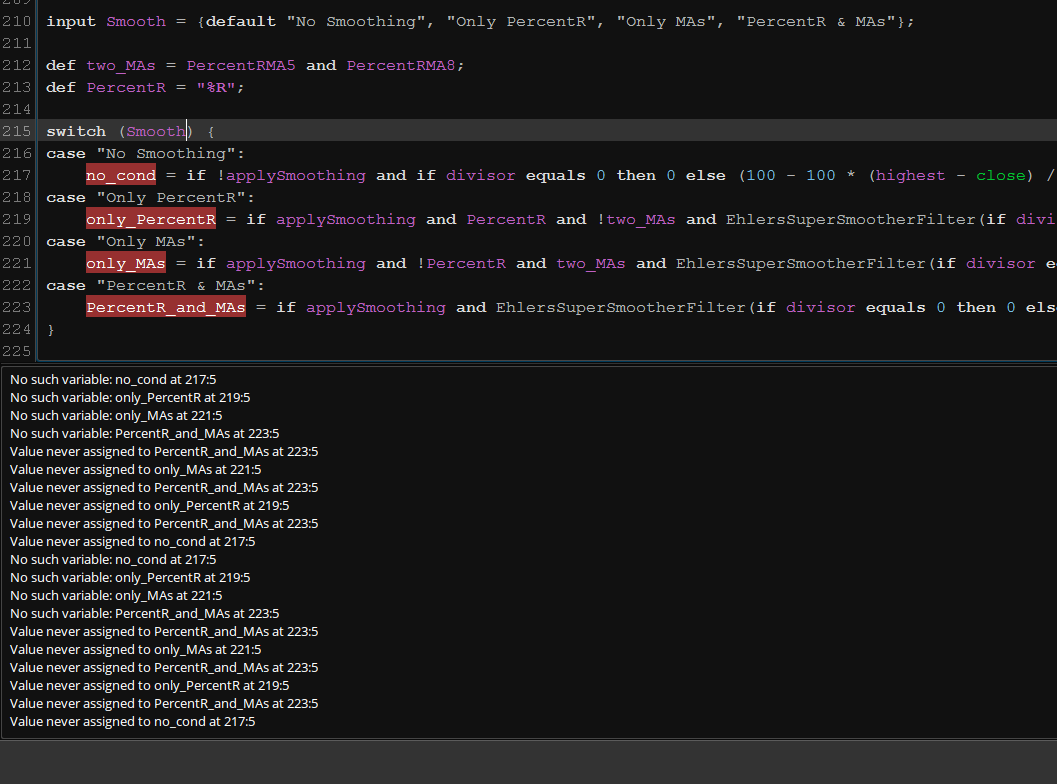Now, as illustrated in the screenshot below, there are no syntax errors, but there is something holding this code back...Once again, I am at a loss to figure this one out:Below is the code for your review, should you choose to accept this missionThanks in advance for your time and effort in this situation...

Code:
``````# filename: MR__EZ_PercentR_MAC__smooth_test_
# idea source: @HighBredCloud and https://usethinkscript.com/threads/moving-average-crossover-rsi-indicator-for-thinkorswim.185/

declare lower;

# Gmode

def lengthRSI = 14;
def price = close;

input averageType = AverageType.WILDERS;

def NetChgAvg = MovingAverage(averageType, price - price, lengthRSI);
def TotChgAvg = MovingAverage(averageType, AbsValue(price - price), lengthRSI);
def ChgRatio = if TotChgAvg != 0 then NetChgAvg / TotChgAvg else 0;

plot RSI = 50 * (ChgRatio + 1);
RSI.SetDefaultColor(CreateColor(0, 102, 204));
RSI.SetLineWeight(3);
#RSI.HideTitle();
RSI.HideBubble();
RSI.Hide();

input n1 = 9; #Channel Length
input n2 = 26; #Average Length
input n3 = 13; #Short length
def multi = no; #Multi-exchange?

def src0 = hlc3;

def src1 = hlc3(period = AggregationPeriod.MONTH);
def src2 = hlc3(period = AggregationPeriod.MONTH);
def src3 = hlc3(period = AggregationPeriod.MONTH);
def src4 = hlc3(period = AggregationPeriod.MONTH);

script tci {

input src = hlc3;
input n1 = 9; #Channel Length
input n2 = 26; #Average Length
plot tci = MovAvgExponential((src - MovAvgExponential(src, n1)) / (0.025 * MovAvgExponential(AbsValue(src - MovAvgExponential(src, n1)), n1)), n2) + 50;
tci.Hide();
}

#mf(src) => rsi(sum(volume * (change(src) <= 0 ? 0 : src), n3), sum(volume * (change(src) >= 0 ? 0 : src), n3))

script mf {

input src = hlc3;
input n3 = 13; #Short length

def rsi = reference RSI();

#plot mf = rsi(Sum(volume * (if Average(src) <= 0 then 0 else src), n3), Sum(volume * (if Average(src) >= 0 then 0 else src), n3));
plot mf = RSI(n3, src);
mf.Hide();
}

script willy {

input src = hlc3;
input n2 = 26; #Average Length
plot willy = 60 * (src - Highest(src, n2)) / (Highest(src, n2) - Lowest(src, n2)) + 80;
willy.Hide();

}

#csi(src) => avg(rsi(src, n3),tsi(src0,n1,n2)*50+50)

script csi {

input src = hlc3;
input n1 = 9; #Channel Length
input n2 = 26; #Average Length
input n3 = 13; #Short length

def rsi = reference RSI();

plot csi = RSI(n3, src) + TrueStrengthIndex(n1, n2) / 2 * 50 + 50;
csi.Hide();

}

script godmode {

input src = hlc3;
def rsi = reference RSI();
plot godmode = (tci(src) + CSI(src) + mf(src) + willy(src)) / 4;
godmode.Hide();

}

input src = hlc3;
input n3 = 13; #Short length

def rsi = reference RSI();

plot tradition = (tci(src) + mf(src) + RSI(n3, src)) / 3;

}

def wt1 = if multi then (godmode(src0) + godmode(src1) + godmode(src2) + godmode(src3) + godmode(src4) / 5) else tradition(src0);

def wt2 = SimpleMovingAvg(wt1, 6);

def extended = if wt2 < 20 then wt2 + 5 else if wt2 > 80 then wt2 - 5 else Double.NaN;

plot wta = wt1;
wta.SetDefaultColor(Color.GREEN);
wta.SetLineWeight(2);
wta.Hide();

plot wtb = wt2;
wtb.SetDefaultColor(Color.RED);
wtb.SetLineWeight(2);
wtb.Hide();

plot ext = extended; #Caution!
ext.SetPaintingStrategy(PaintingStrategy.POINTS);
ext.SetDefaultColor(Color.YELLOW);
ext.SetLineWeight(3);

# PercentR_MAC

input length = 14;
input over_Bought = 80;
input over_Sold = 20;
input showBreakoutSignals = yes;
input PercentRMALength5 = 5; #hint rsiMALength: RSI Moving Average Length
input PercentRMALength8 = 8;
input PercentRAverageType = AverageType.EXPONENTIAL;
input applySmoothing = yes; #Smooth PercentR?
input lowBand = 10; #Smoothing LowerBand
input data = close;
input lower = low; #Research Lower = Low?

def highest = Highest(high, length);
def divisor = highest - Lowest(low, length);

def PI = 3.14159265359;
def a1 = Exp(-PI * Sqrt(2) / lower);
def coeff2 = 2 * a1 * Cos(Sqrt(2) * PI / lower);
def coeff3 = - Power(a1, 2);
def coeff1 = 1 - coeff2 - coeff3;
def filt = coeff1 * (data + (data)) / 2 + coeff2 * (filt) + coeff3 * (filt);
def rough_it = if divisor equals 0 then 0 else (100 - 100 * (highest - close) / divisor);

# plot and smooth PercentR
plot "%R" = if applySmoothing then EhlersSuperSmootherFilter(if divisor equals 0 then 0 else 100 - 100 * (highest - close) / divisor, lowBand) else rough_it;
"%R".DefineColor("OverBought", GetColor(9));
"%R".DefineColor("Normal", GetColor(7));
"%R".DefineColor("OverSold", GetColor(1));
"%R".AssignValueColor(if "%R" > over_Bought then "%R".Color("OverBought") else if "%R" < over_Sold then "%R".Color("OverSold") else "%R".Color("Normal"));
"%R".SetLineWeight(3);

# plot the PercentR Moving Averages
def PercentRMA5 = MovingAverage(PercentRAverageType, "%R", PercentRMALength5);
plot PercentRMovAvg5 = PercentRMA5;
PercentRMovAvg5.SetDefaultColor(Color.GREEN);
PercentRMovAvg5.SetLineWeight(3);

def PercentRMA8 = MovingAverage(PercentRAverageType, "%R", PercentRMALength8);
plot PercentRMovAvg8 = PercentRMA8;
PercentRMovAvg8.SetDefaultColor(Color.RED);
PercentRMovAvg8.SetLineWeight(3);

plot OverBought = over_Bought;
OverBought.SetDefaultColor(Color.DARK_RED);
OverBought.HideTitle();

plot OverSold = over_Sold;
OverSold.SetDefaultColor(Color.DARK_GREEN);
OverSold.HideTitle();

plot fifty_line = 50;
fifty_line.SetDefaultColor(GetColor(8));
fifty_line.HideTitle();
fifty_line.SetStyle(Curve.SHORT_DASH);

# plot the Breakout Signals
plot UpSignal = if "%R" crosses above OverSold then OverSold else Double.NaN;
UpSignal.SetHiding(!showBreakoutSignals);
UpSignal.SetDefaultColor(Color.UPTICK);
UpSignal.SetPaintingStrategy(PaintingStrategy.ARROW_UP);
UpSignal.SetLineWeight(3);
UpSignal.HideTitle();

plot DownSignal = if "%R" crosses below OverBought then OverBought else Double.NaN;
DownSignal.SetHiding(!showBreakoutSignals);
DownSignal.SetDefaultColor(Color.DOWNTICK);
DownSignal.SetPaintingStrategy(PaintingStrategy.ARROW_DOWN);
DownSignal.SetLineWeight(3);
DownSignal.HideTitle();

input Smooth = {default "No Smoothing", "Only PercentR", "Only MAs", "PercentR & MAs"};

def two_MAs = PercentRMA5 and PercentRMA8;
def PercentR = "%R";

plot cond;

switch (Smooth) {
case "No Smoothing":
cond = if !applySmoothing and if divisor equals 0 then 0 else (100 - 100 * (highest - close) / divisor) then 1 else 0;
case "Only PercentR":
cond = if applySmoothing and PercentR and !two_MAs and EhlersSuperSmootherFilter(if divisor equals 0 then 0 else 100 - 100 * (highest - close) / divisor, lowBand) then 1 else 0;
case "Only MAs":
cond = if applySmoothing and !PercentR and two_MAs and EhlersSuperSmootherFilter(if divisor equals 0 then 0 else 100 - 100 * (highest - close) / divisor, lowBand) then 1 else 0;
case "PercentR & MAs":
cond = if applySmoothing and EhlersSuperSmootherFilter(if divisor equals 0 then 0 else 100 - 100 * (highest - close) / divisor, lowBand) then 1 else 0;
}``````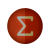Case: value never assigned to error Questions 1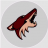How do I plot only the latest value of a variable? Questions 3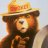How do I obtain the HI or LOW value of a Premarket time frame? Questions 2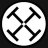Average candlestick value? Questions 0